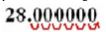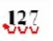Ontario Tech acknowledges the lands and people of the Mississaugas of Scugog Island First Nation.

We are thankful to be welcome on these lands in friendship. The lands we are situated on are covered by the Williams Treaties and are the traditional territory of the Mississaugas, a branch of the greater Anishinaabeg Nation, including Algonquin, Ojibway, Odawa and Pottawatomi. These lands remain home to many Indigenous nations and peoples.

We acknowledge this land out of respect for the Indigenous nations who have cared for Turtle Island, also called North America, from before the arrival of settler peoples until this day. Most importantly, we acknowledge that the history of these lands has been tainted by poor treatment and a lack of friendship with the First Nations who call them home.

This history is something we are all affected by because we are all treaty people in Canada. We all have a shared history to reflect on, and each of us is affected by this history in different ways. Our past defines our present, but if we move forward as friends and allies, then it does not have to define our future.

# Basic Unit Conversions

The ability to convert between different units is an important aspect of math which is necessary for success in all of your science courses! For most science courses, you will be expected to know how to perform conversions between units with prefixes ranging from pico to mega. These larger and smaller units are defined in certain powers of 10, so making conversions is easy.

However, before we can convert between units, we need to know what each prefix represents. The following table provides the prefix, symbol, and meaning of the required units.

 Prefix Symbol Multiplier Mega M 106 Kilo K 103 Centi C 10-2 Milli M 10-3 Micro μ 10-6 Nano N 10-9 Pico P 10-12

## CONVERTING FROM ONE UNIT TO ANOTHER

There are two common methods used for unit conversion.

### METHOD 1

• Subtract the power of the multiplier corresponding to the 2nd unit from that corresponding to the 1st unit.
• Move the decimal the number of places found in step one.
• If step one gives a positive result, the decimal moves to the right (this happens when we go from a larger prefix to a smaller one).
• If step one gives a negative result, the decimal moves to the left (this happens when we go from smaller prefix to larger one).

Example:

Convert 28 kg to mg.

Solution:

In order to convert 28 kg into mg, we would subtract the power of the multiplier for the 2nd unit (-3, since milligrams means we have 10-3 grams) from the first (3, since kilograms means we have 103 grams).

3 – (-3) = 6

Therefore, the decimal is moved 6 places to the right.Answer: 28 000 000 mg, which can be rewritten as 2.8 x 107 mg in scientific notation.

Example:

Convert 127 nm to μ m.

Solution:

In order to convert 127 nm to μ m, we would subtract the power of the multiplier for the 2nd unit (-6, since micrometres means we have 10-6 metres) from the first (-9, since nanometers means we have 10-9 metres).

-9 – (-6)= -3

Therefore, the decimal is moved 3 places to the left.Answer: 0.127 μ m, which can be rewritten as 1.27 x 10-1 μ m in scientific notation.

### METHOD 2

The second method is fairly similar to the first method. The number being converted is multiplied by a power of 10, which is determined by subtracting the power of the multiplier of the second unit from that of the first unit. The difference determines the power by which the value will be multiplied.

Example:

Convert 326 μ g to kg.

Solution:

Subtract the second multiplier’s power from that of the first (3, since kilograms means we have 103 grams, is subtracted from -6, since micrograms means we have 10-6 grams).

(-6) -3= -9

So, to perform the unit conversion, we multiply by 10-9.

Answer: 326 x 10-9kg, which can be written as 3.26 x 10-7 kg in scientific notation.

## CONVERTING MORE THAN ONE SET OF UNITS

More advanced conversions often require two different units to be converted. The best method is to convert one unit at a time.

Example:

Convert 18 km/hr into m/s.

Solution:

18 km/hr x 1000m/km x 1hr/60min x 1min/60sec = 5m/s

Answer: Therefore 18 km/hr = 5 m/s